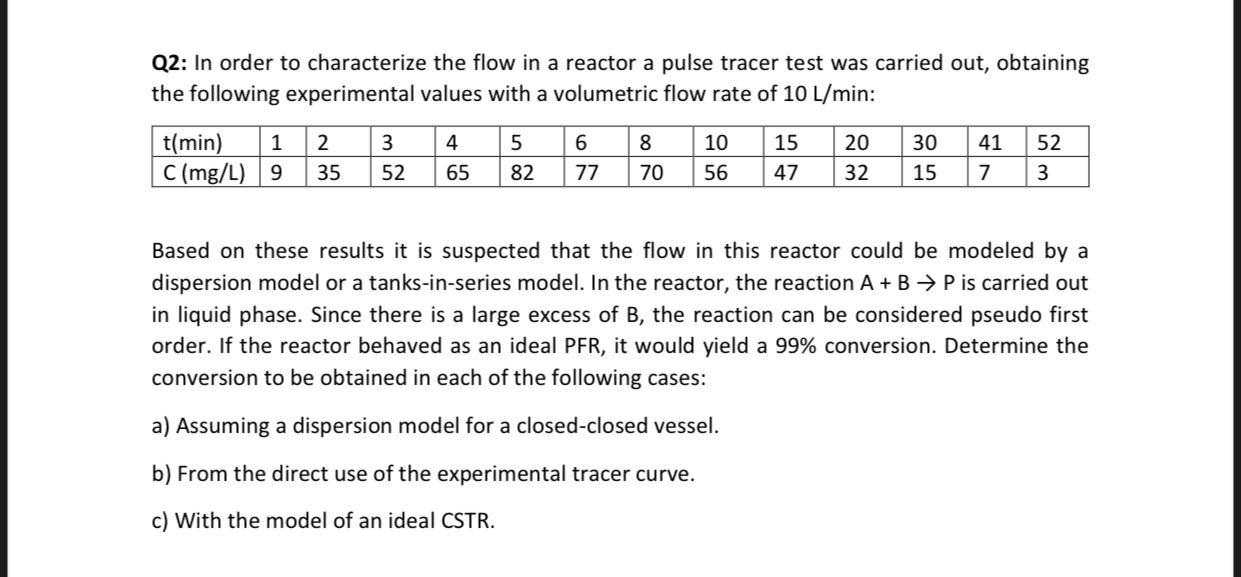# Question I need the answer as soon as possible Please. Q2: In order to characterize the flow in a reactor a pulse tracer test was carried out, obtaining the following experimental values with a volumetric flow rate of 10 L/min: t(min) 1 2 3 4 5 6 8 10 15 20 30 41 52 C (mg/L) | 9 35 52 65 82 77 70 56 47 32 15 7 3 Based on these results it is suspected that the flow in this reactor could be modeled by a dispersion model or a tanks-in-series model. In the reactor, the reaction A + B + P is carried out in liquid phase. Since there is a large excess of B, the reaction can be considered pseudo first order. If the reactor behaved as an ideal PFR, it would yield a 99% conversion. Determine the conversion to be obtained in each of the following cases: a) Assuming a dispersion model for a closed-closed vessel. b) From the direct use of the experimental tracer curve. c) With the model of an ideal CSTR.DMK0T4 The Asker · Chemical Engineering